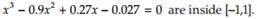### Create an Account

Home / Questions / Roots of a Graphically locate the roots, and determine if a root is simple or of higher ...

# Roots of a Graphically locate the roots, and determine if a root is simple or of higher multiplicity b Approximate the root with higher multiplicity by executing the user-defined function Newton

Roots ofa. Graphically locate the roots, and determine if a root is simple or of higher multiplicity,

b. Approximate the root with higher multiplicity by executing the user-defined function Newton Mod (Section 3.5), and the simple root by executing Newton. Use default parameter values, and let x1 be the closest integer on the left of the root.

Jul 22 2020 View more View LessSubscribe To Get Solution